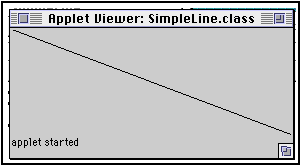# Drawing Lines

Drawing straight lines with Java is easy. Just call

```g.drawLine(x1, y1, x2, y2) ```

where `(x1, y1)` and `(x2, y2)` are the endpoints of your lines and `g` is the `Graphics` object you're drawing with.

This program draws a line diagonally across the applet.

``````import java.applet.*;
import java.awt.*;

public class SimpleLine extends Applet {

public void paint(Graphics g) {

g.drawLine(0, 0, this.getSize().width, this.getSize().height);

}

}``````
Here's the resultCopyright 1997, 1998 Elliotte Rusty Harold
elharo@metalab.unc.edu